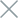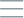• Home
• Tags
• Series
•# Binary Search Algorithm in PHP: Explanation, Steps and Example

The Binary Search algorithm is an efficient method to find a specific value in a sorted array. This approach divides the array into smaller parts and continuously compares the value at the middle position of the search range with the target value. If the values match, the desired value is found; otherwise, the algorithm continues to narrow down the search range and repeats the process until the value is found or no more elements are left to examine.

Steps:

1. Initialize the search range: Begin by selecting the search range from the first position `left` to the last position `right` of the array.
2. Find the middle point: Calculate the middle point by averaging the `left` and right positions; this is the middle point of the search range.
3. Compare values: Compare the value at the middle point with the target value.
4. Handle comparison result: If the value at the middle point matches the target value, return this position. If the value at the middle point is less than the target value, update the left position to middle + 1 to search the right half. If the value at the middle point is greater than the target value, update the right position to middle - 1 to search the left half.
5. Repeat: Repeat steps 2 to 4 until the value is found or there are no more elements to check `left > right`.

• Efficient performance: The time complexity of the algorithm is O(log n), making it highly efficient for handling large datasets.
• Effective for large datasets: Binary Search is effective in reducing the number of elements to examine quickly for large datasets.

• Applicable only to sorted arrays: The algorithm only works on sorted arrays.
• Variable number of steps: The number of steps required to find the value depends on its position in the array, and it can take many steps for values near the ends.

## Example: Binary Search for finding the value 12 in a sorted array in PHP

``````function binarySearch(\$arr, \$target) {
\$left = 0;
\$right = count(\$arr) - 1;

while (\$left <= \$right) {
\$mid = floor((\$left + \$right) / 2);

if (\$arr[\$mid] == \$target) {
return \$mid; // Return the position of the value
} elseif (\$arr[\$mid] < \$target) {
\$left = \$mid + 1;
} else {
\$right = \$mid - 1;
}
}

}

\$array = [2, 5, 8, 12, 15, 20, 30];
\$targetValue = 12;

\$result = binarySearch(\$array, \$targetValue);

if (\$result != -1) {
echo "Value \$targetValue found at position \$result.";
} else {
1. We start with the initial search range from the first position `left = 0` to the last position `right = 6` of the array.
2. We calculate the middle point (mid) by averaging the left and right positions; `mid = 3`. The value at mid is 12.
3. We compare the value at `mid (12`) with the target value (12) and find a match, so we return position 3.